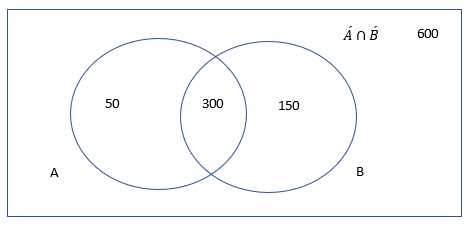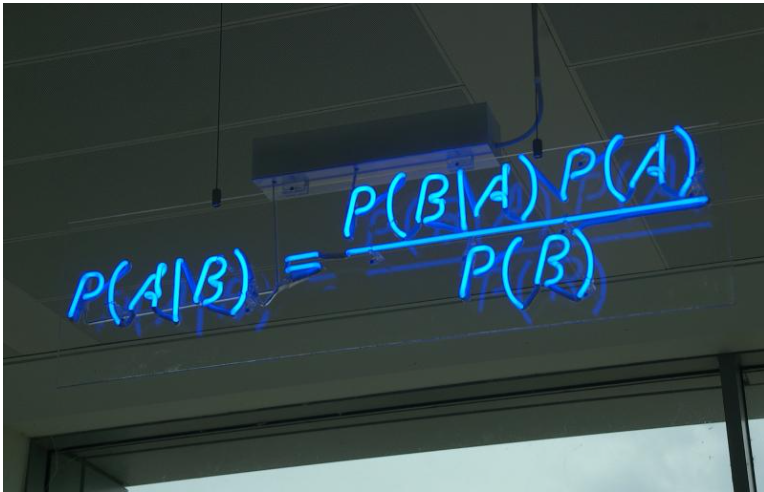#### Naive BayesAnalytics Vidhya

Naive Bayes is a probabilistic machine learning algorithm. It is used widely to solve the classification problem. In addition to that this algorithm works perfectly in natural language problems…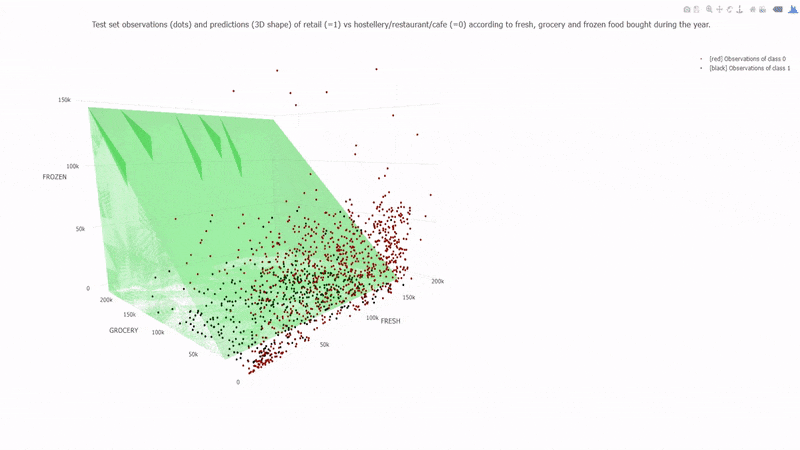Towards Data Science

Naive Bayes Data Science Machine learning Artificial Intelligence Deep learning neural networks algorithms data datasets

Read more at Towards Data Science#### 1.9. Naive BayesScikit-learn User Guide

Naive Bayes methods are a set of supervised learning algorithms based on applying Bayes’ theorem with the “naive” assumption of conditional independence between every pair of features given the val......

Read more at Scikit-learn User Guide#### The Not So Naive BayesTowards Data Science

Have you ever wondered how your email service provider classifies a mail as spam or not spam almost immediately after you have received it? Or have you thought how the recommendations by online…

Read more at Towards Data Science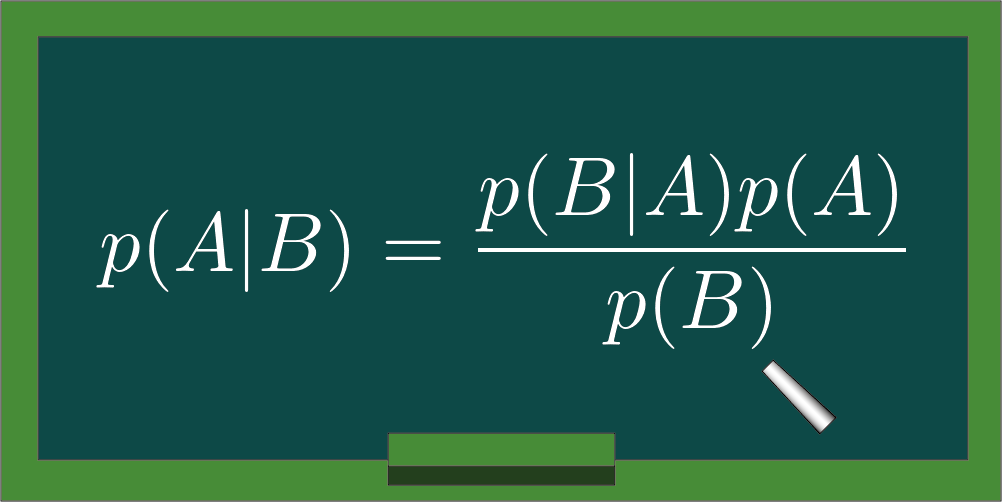#### Naive Bayes ExplainedTowards Data Science

Machine learning approaches for classification can be discriminative and generative in nature. Basically, the distinction between one and the other lies in the process that is followed to obtain…

Read more at Towards Data Science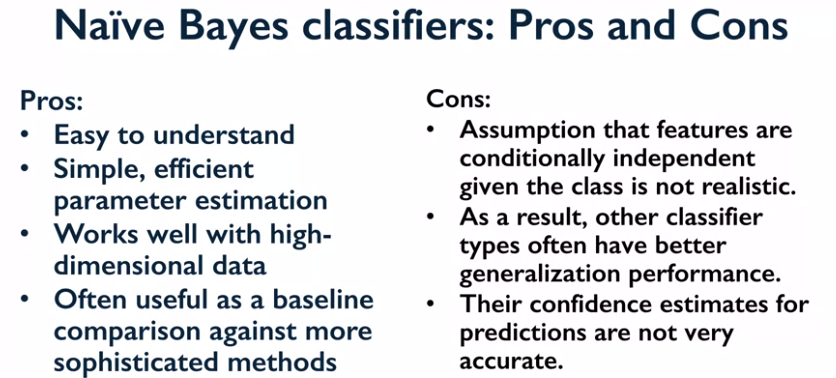#### Naïve Bayes ClassifiersAnalytics Vidhya

Naive Bayes classifiers are a family of classifiers based in Bayes’ theorem that are quite similar to the linear models. However, they tend to be even faster in training. The price paid for this…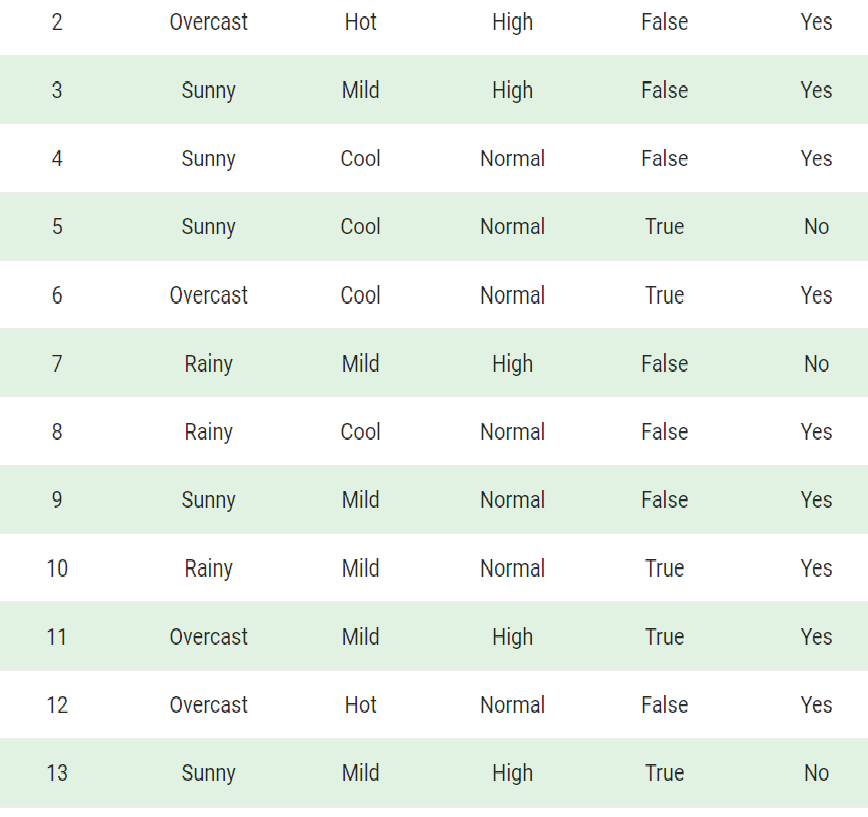#### Naive Bayes ClassifiersTowards AI

Naive Bayes classifiers are a collection of classification algorithms based on Bayes’ Theorem. It is not a single algorithm, but a family of algorithms where all of them share a common principle…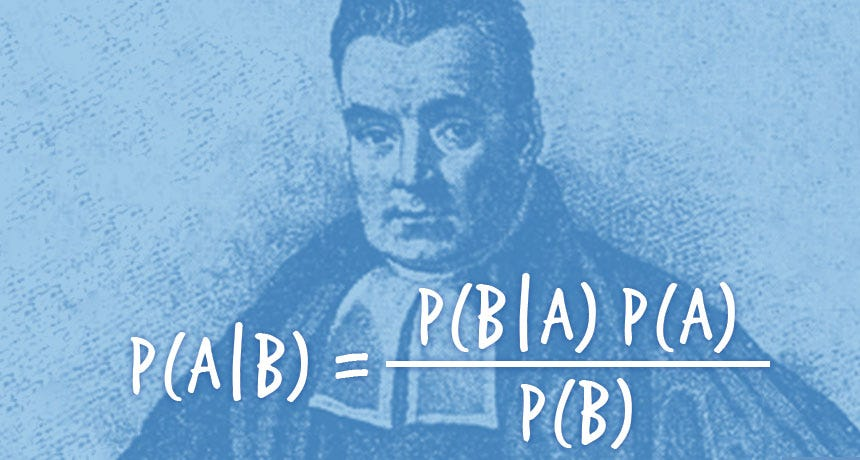#### The Naive Bayes ClassifierTowards Data Science

The Naïve Bayes Classifier is perhaps the simplest machine learning classifier to build, train, and predict with. This post will show how and why it works. Part 1 reveals that the much-celebrated…

Read more at Towards Data Science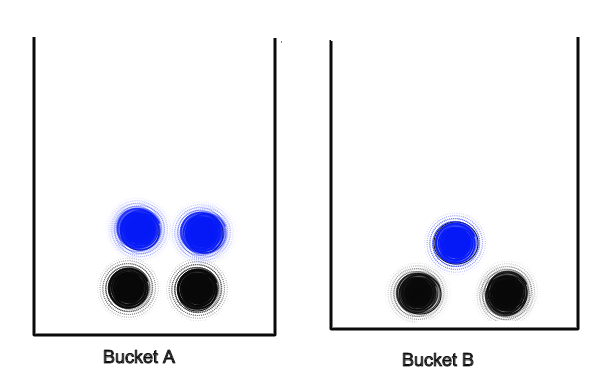#### Naive Bayes ClassificationAnalytics Vidhya

Naive Bayes is a supervised learning classification. It is a probabilistic classifier based on Bayes theorem. The name naive stems from the fact that classifier assumes that pairs of features are…#### Naive Bayes in Machine LearningTowards Data Science

Bayes’ theorem finds many uses in the probability theory and statistics. There’s a micro chance that you have never heard about this theorem in your life. Turns out that this theorem has found its…

Read more at Towards Data Science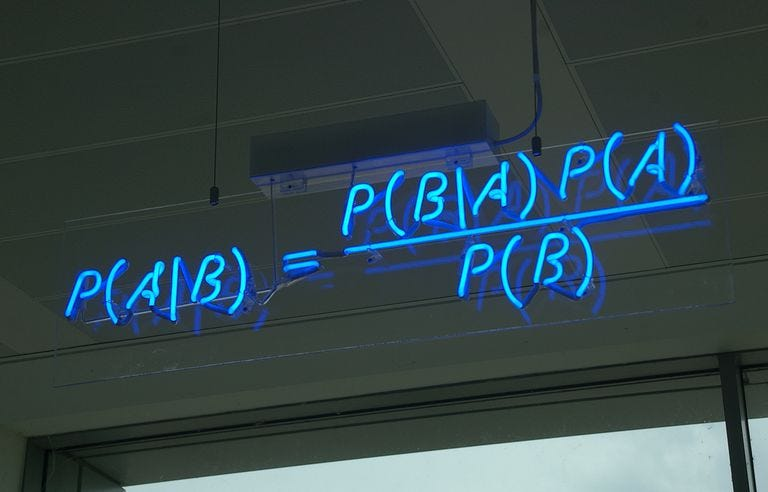#### The Naivety of Naive BayesAnalytics Vidhya

What the developers wanted to achieve through Machine Learning was to enable computers to have minds of their own and learn to make decisions by themselves. Such an objective, as we know by the…#### Naive BayesDive intro Deep Learning Book

Throughout the previous sections, we learned about the theory of probability and random variables. To put this theory to work, let’s introduce the naive Bayes classifier. This uses nothing but probabi...

Read more at Dive intro Deep Learning Book#### Machine Learning 101 - Naive Bayes(2)Towards Data Science

This the second part of my series of Machine Learning 101, I had tried to explain Decision Tree and Entropy Calculation at the very first post of my series. Here is the link: Machine Learning 101…

Read more at Towards Data Science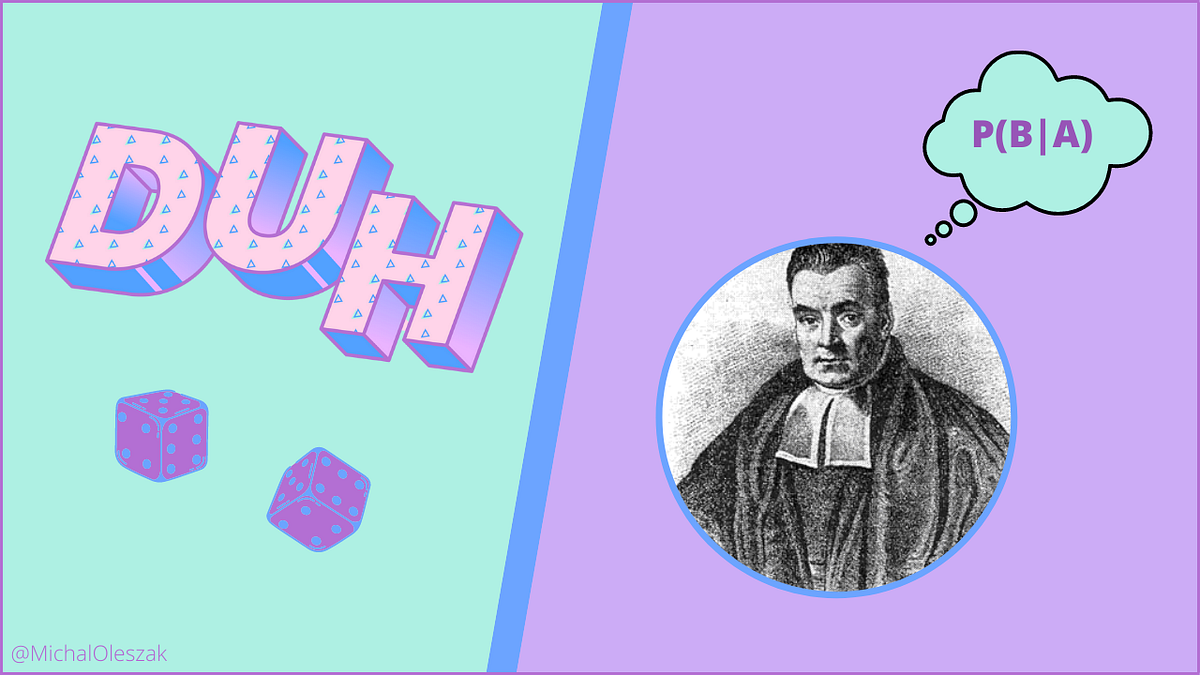#### Not-so-naive BayesTowards Data Science

Improve the simple Bayesian classifier by releasing its naive assumption. Continue reading on Towards Data Science

Read more at Towards Data Science#### Cat or Dog? Introduction to Naive BayesTowards Data Science

Introduction to the Naive Bayes classification algorithm

Read more at Towards Data Science#### Naive Bayes for Machine LearningMachine Learning Mastery

Last Updated on August 15, 2020 Naive Bayes is a simple but surprisingly powerful algorithm for predictive modeling. In this post you will discover the Naive Bayes algorithm for classification. After ...

Read more at Machine Learning Mastery#### Back to Basics: Naive Bayes DemystifiedTowards Data Science

Our goal is to learn about Naïve Bayes and apply it to a real-world problem, spam detection. Why Naïve Bayes? Consider building an email spam filter using machine learning. You would like to…

Read more at Towards Data Science#### Naive Bayes Tutorial for Machine LearningMachine Learning Mastery

Last Updated on August 12, 2019 Naive Bayes is a very simple classification algorithm that makes some strong assumptions about the independence of each input variable. Nevertheless, it has been shown ...

Read more at Machine Learning Mastery

#### Naive Bayes AlgorithmAnalytics Vidhya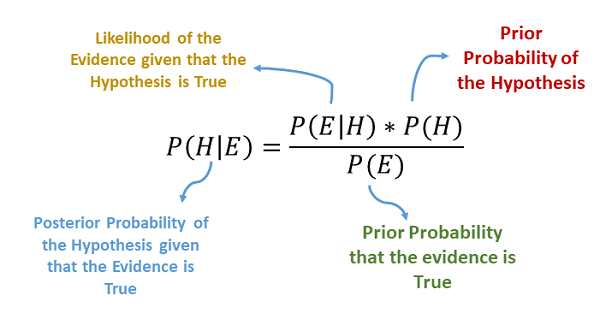#### Naïve Bayes AlgorithmAnalytics Vidhya

Naive Bayes is a classification technique that is based on Bayes’ Theorem with an assumption that all the features that predicts the target value are independent of each other. It calculates the…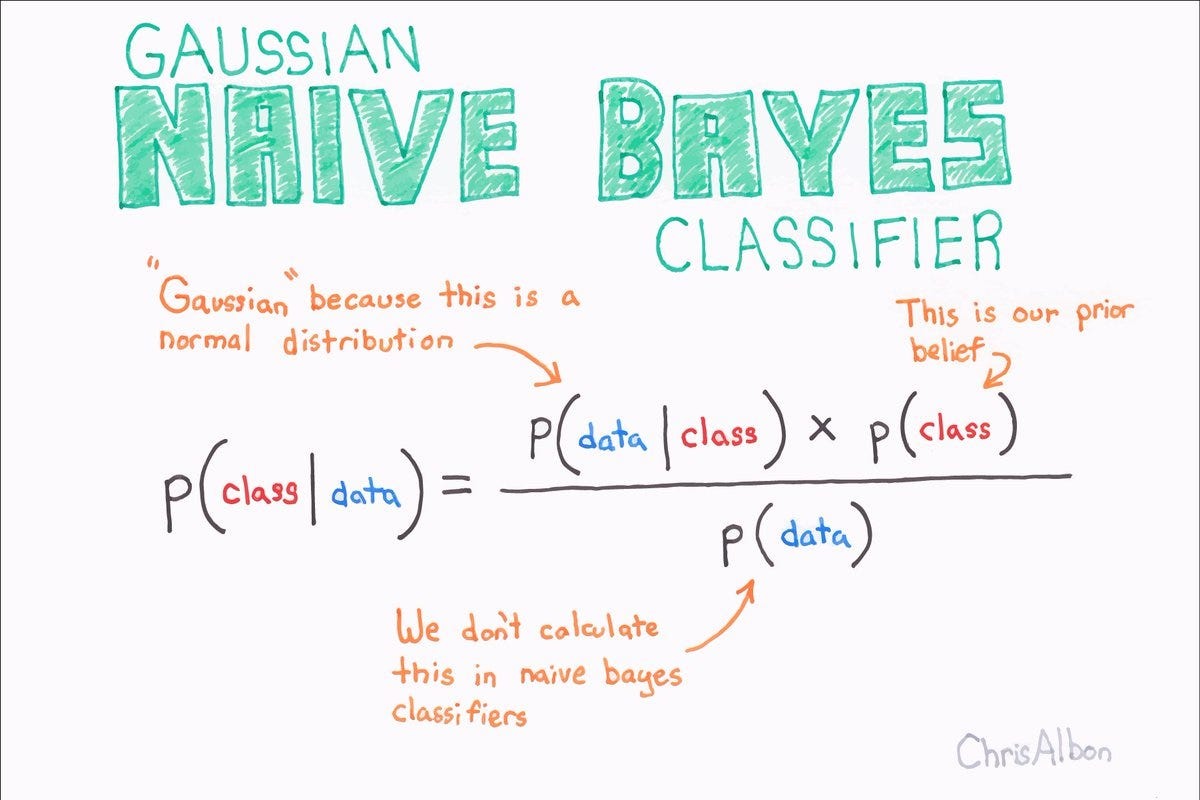#### Naive Bayes TheoremBecoming Human: Artificial Intelligence Magazine

Bayes theorem is one of the earliest probabilistic inference algorithms developed by Reverend Bayes (which he used to try and infer the existence of God no less) and still performs extremely well for…...

Read more at Becoming Human: Artificial Intelligence Magazine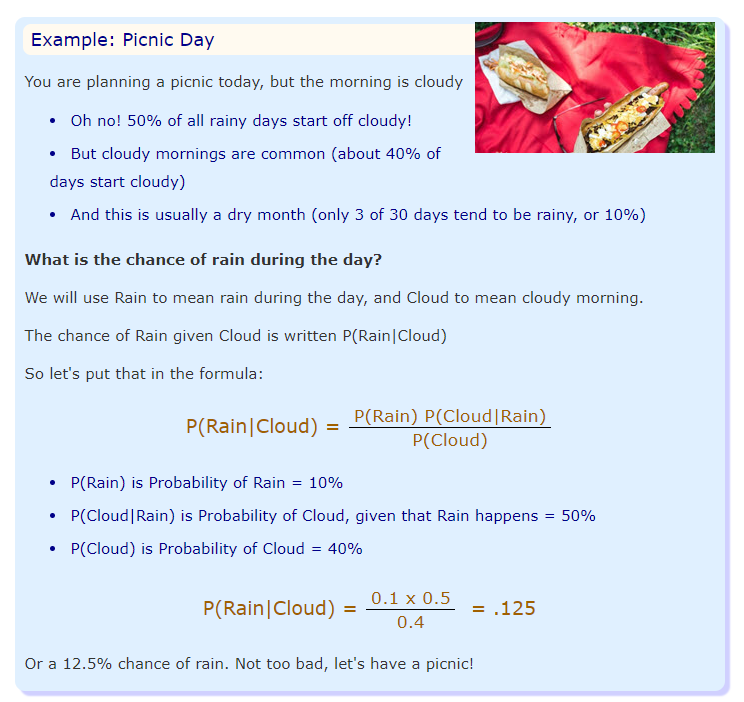#### Introduction to Naive Bayes for Machine LearningAnalytics Vidhya

Naive Bayes is a family of probabilistic algorithms that take advantage of probability theory and Bayes’ Theorem. They are probabilistic, which means that they calculate the probability of each tag…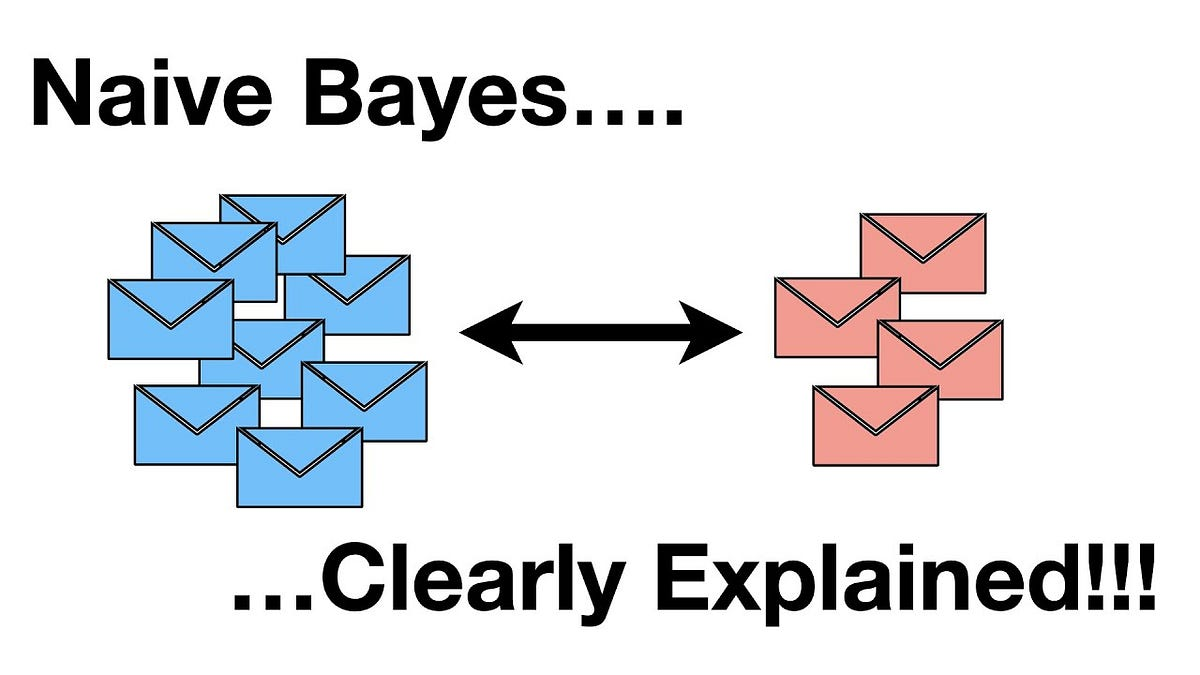#### All you need to know about Naive BayesAnalytics Vidhya

In this blog I will be writing about simple, yet effective and commonly used, machine learning classifier, that is, Naive Bayes. Here I will explain about What is Naive Bayes, Bayes theorem and its…